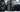Theophilus Ngaribvume

This is Where i Share my Experiences, Ideas, and Thoughts. One Post a Day.

Introduction to theory of computation

19 March, 2020 - 3 min readThis post seeks to introduce the reader to what theory of computation is all about and how computers can be better understood on a fundamental level.

Are there problems that a computing machine cannot solve?, no matter:

• The superiority of the algorithm
• The programming language used
• The hardware we use

If the problems exist, how do we identify them.

These questions are answered in theory of computation.

Branches of Theory of Computation

1. Complexity theory - A theory of problem classification, the easy problems and the hard problems.
2. Computability theory - A theory on how to classify problems into intractable and tractable problems.
3. Automata theory - A theory that studies abstract computing machines, the definitions and attributes of different computation models.

• Finite Automata - Are useful models used in different kinds of hardware and software.
• Software for regulating the behavior of circuits
• Lexical analyzer for compilers
• Text processors
• Context Free Grammars - Models for defining programming languages.
• Turing Machines - An abstract model of a computer e.g a PC.

Introduction to Theory of Computation Solutions

1. Pattern recognition in text i.e string searching algorithms.
2. Identifying tractable and intractable problems
3. Compiler design
4. Spell checkers
5. Evaluating arithmetic problems
6. Implementation of genetic programming
7. Implementation of neural networks
8. Implementation of robotics problems

Finite Automata

Some systems can be viewed as being in one of a finite number of states. The state is used to remember the important part of the history. Finite automata allows the implementation of systems with finite computing resources.

A typical example is an off/on switch. The device must remember it's state, off or on and allows a user to change the state depending on the current switch state.

It is a method for defining languages. This model is said to be finite because the possible states and number of alphabet letters are finite. The changes in state is determined by input.

Finite Automata has:

• Finite set of state, initial state and final states
• An alphabet of possible input symbols
• Finite set of transitions that takes a state and symbol of input and gives the state to go to next.

Deterministic Finite State Automaton

A deterministic finite automaton is an abstract device where one can determine the state to which the machine will move for each input string. It is a device that:

• Has one initial state
• At least one accept state
• Always in one of a finite number of state
• Is attached to an input stream
• Capable of switching state which is uniquely determined by the current state and symbol read from the input.